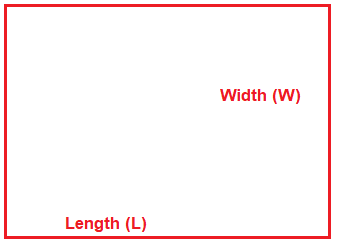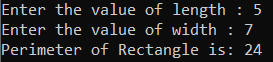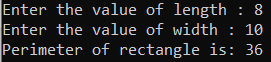# Perimeter of Rectangle in C#

## Calculate Perimeter of Rectangle in C# with Examples

In this article, I am going to discuss how to Calculate the Perimeter of a Rectangle in C# with Examples. Please read our previous article, where we discussed how to Convert Inches to Centimeters in C#. Here, in this article, first, we will discuss what is Rectangle, then we will discuss how to Calculate the Perimeter of a Rectangle and finally, we will see how to implement the Perimeter of a Rectangle Program in C#.

##### What is Rectangle?

A Rectangle is a four sided-polygon, having all the internal angles equal to 90 degrees. The two sides at each corner or vertex meet at right angles. The opposite sides of the rectangle are equal in length which makes it different from a square. A rectangle is characterized by length (L) and width (W). Both length and width are different in size.##### Formula to Calculate Perimeter of Rectangle.

The perimeter of a rectangle is the total length of all the sides of the rectangle. Hence, we can find the perimeter by adding all four sides of a rectangle.
The perimeter of the given rectangle is l + w + l + w.
Perimeter = (2 * l) + (2 * w) [Opposite sites of rectangle are equal]
Perimeter = 2 (l + w) [taking common 2]

##### Algorithm to Calculate the Perimeter of Rectangle in C#

The following are the steps that we need to follow to solve this problem.

1. Take a variable named length and store the length of the rectangle into this.
2. Take another variable named width and store the width of the rectangle into this.
3. Take the last variable named perimeter and store the result of the calculation.
4. Perimeter = 2 * (l + w);
##### Example:

The following C# Program will calculate the Perimeter of the Rectangle.

```using System;
public class PerimeterOfRectangle
{
public static void Main()
{
Console.Write("Enter the value of length : ");

Console.Write("Enter the value of width : ");

decimal Perimeter = 2 * (Length + Width);

Console.Write("Perimeter of Rectangle is: " + Perimeter);
}
}
```
###### Output:##### C# Code (Using function)

In the below C# example, we have created one method to calculate the perimeter of the Rectangle.

```using System;
public class PerimeterOfRectangle
{
public static void Main()
{
Console.Write("Enter the value of length : ");

Console.Write("Enter the value of width : ");

PerimeterOfRectangle obj = new PerimeterOfRectangle();
Console.Write("Perimeter of rectangle is: " + obj.CalculatePerimeterOfRectangel(length, width));
}

decimal CalculatePerimeterOfRectangel(decimal length, decimal width)
{
decimal perimeter = 2 * (length + width);
return perimeter;

//Or the same above 2 lines of code can be replaced with
/******* return (2* (length + width)); **********/
}
}
```
###### Output:In the next article, I am going to discuss Calculate Perimeter of Square in C# with Examples. Here, in this article, I try to explain how to Calculate the Perimeter of the Rectangle in C# with Examples and I hope you enjoy this article.